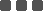### Description

Companies are sometimes faced with the possibility of catastrophic accident consequences and need to assess their likelihood to determine what remediation may be necessary. In such cases, the likelihood of an accident occurring is usually very low and must be determined through probability modeling. Probability modeling techniques such as fault tree and event tree analysis are used to assess the likelihood of these process accidents and the reliability of process systems. These analyses combine the probabilities of the events that make up the accident scenario. The resulting event probabilities and frequencies are also used as input into quantitative risk analysis and reliability studies.

Both historical incident data and failure rate data can be used to quantify fault and event trees and evaluate incident probabilities. The studies may involve dependent failure analysis to account for common cause failures and human reliability analysis to quantify human error probabilities. Uncertainty analysis is used to explore the effects of data uncertainties on the modeling results and importance and sensitivity analysis help to identify the most important and significant contributors.

### Why Model Probabilities?

Increasingly, companies recognize that reliance on purely qualitative analysis is insufficient where high consequence, low probability accidents are concerned. Qualitative probability estimates are subjective. Quantitative analysis provides a sounder basis for decision making on the tolerability of catastrophic accident probabillities.

Often, the first step in quantitative analysis is to calculate the probability of an accident rather than calculate its consequences. Uncertainties in accident probabilities are generally greater than uncertainties in accident consequences and quantitative analysis makes them more apparent so they can be addressed more readily in decision making. Also, it is easier to calculate accident probabilities than accident consequences.

### Why Primatech?

Primatech performs event and fault tree analysis to calculate the frequencies and probabilities of events. We have compilations of failure rate data that are available for use in these analyses. Also, Primatech is experienced in teaching others to perform these analyses.

### Services Offered

• Event tree analysis.

We calculate the frequencies of key event sequences for a process and provide a report that documents the results.

• Fault tree analysis. We calculate the probabilities of important fault tree top events and use them with event tree analysis to determine overall hazard scenario frequencies. We provide a report that documents the results.

• Sensitivity, importance and uncertainty analysis.

We determine the input variables to which the results of event and fault tree analysis are most sensitive. Also, we assess which variables play the most important role in determining the results of the analysis. We propagate uncertainties in the input variables through the analyses to determine the uncertainties in the calculated results. We provide a report that documents the calculations.